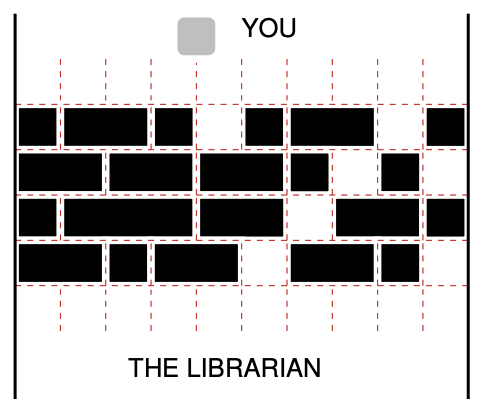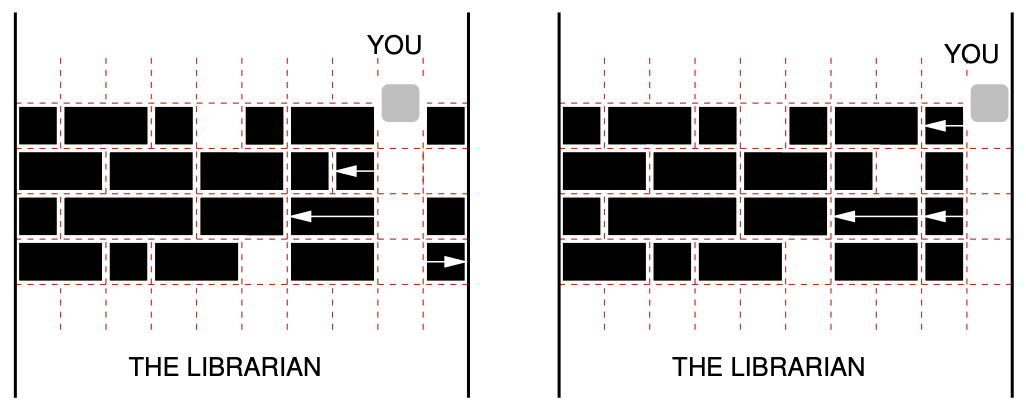시간 제한 메모리 제한 제출 정답 맞은 사람 정답 비율
1 초 512 MB 0 0 0 0.000%

## 문제

You are in a library equipped with bookracks that move on rails. There are many parallel rails, i.e., the bookracks are organized in several rows, see figure:The boockracks in the library. There is no passage to the librarian at the moment.

To borrow a book, you have to find the librarian, who seems to hide on the opposite side of the bookracks. Your task then is to move the racks along the rails so that a passage forms. Each rack has a certain integer width, and can be safely positioned at any integer point along the rail. (A rack does not block in a non-integer position and could accidentally move in either direction). The racks in a single row need not be contiguous — there can be arbitrary (though integer) space between two successive bookracks. A passage is formed at position k if there is no bookrack in the interval (k, k + 1) in any row (somehow you don’t like the idea of trying to find a more sophisticated passage in this maze.)The passages formed in the library: at position 8 (the left figure) and at position 9 (the right figure). Both attained at cost 3 by moving the bookracks marked with arrows.

Moving a rack requires a certain amount of effort on your part: moving it in either direction costs 1. This cost does not depend on the distance of the shift, which can be explained by a well known fact that static friction is considerably higher than kinetic friction. Still, you are here to borrow a book, not to work out, so you would like to form a passage (at any position) with as little effort as possible.

## 입력

The input contains several test cases. The first line of the input contains a positive integer Z ≤ 15, denoting the number of test cases. Then Z test cases follow.

Two space separated integers R and L (1 ≤ R, 1 ≤ L ≤ 106) are given in the first line of an input instance. They denote the number of rows and the width of each and every row, respectively. Then R lines with rows descriptions follow. Each such line starts with an integer ni, followed by ni integers ai,1, ai,2,. . . ai,ni, all separated by single spaces. Number ai,j denotes either the width of a bookrack when ai,j > 0 or a unit of empty space when ai,j = 0. Note that for any row i, Σjai,j equals L minus the number of ai,j that are equal to zero. You may assume that n1 + n2 + · · · + nR ≤ 2 × 107. Moreover, there will be at least one 0 in the description of each row, which means that creating a passage is always possible.

## 출력

In the first line, your program should output the minimum cost of making a passage through the bookracks. In the second line, it should print out the increasing sequence of all the positions at which a minimum cost passage can be formed.

## 예제 입력 1

1
4 10
8 1 2 1 0 1 2 0 1
7 2 2 2 1 0 1 0
6 1 3 2 0 2 1
7 2 1 2 0 2 1 0


## 예제 출력 1

3
8 9